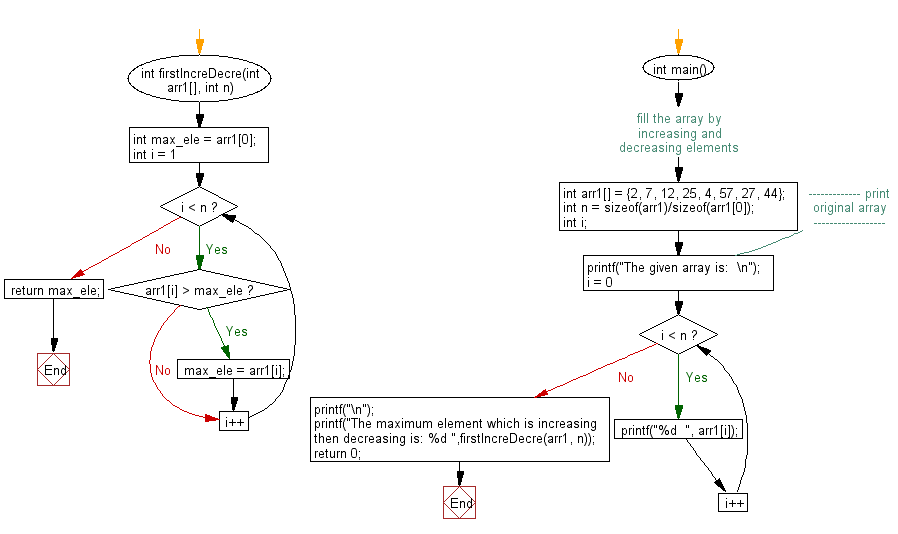﻿ C exercises: Find the maximum element in an array which is first increasing and then decreasing - w3resource# C Exercises: Find the maximum element in an array which is first increasing and then decreasing

## C Array: Exercise-87 with Solution

Write a program in C to find the maximum element in an array which is first increasing and then decreasing.

Sample Solution:

C Code:

``````#include <stdio.h>

int firstIncreDecre(int arr1[], int n)
{
int max_ele = arr1;
for (int i = 1; i < n; i++)
{
if (arr1[i] > max_ele)
max_ele = arr1[i];
}
return max_ele;
}

int main()
{
int arr1[] = {2, 7, 12, 25, 4, 57, 27, 44}; //fill the array by increasing and decreasing elements
int n = sizeof(arr1)/sizeof(arr1);
int i;
//------------- print original array ------------------
printf("The given array is:  \n");
for(i = 0; i < n; i++)
{
printf("%d  ", arr1[i]);
}
printf("\n");
//-----------------------------------------------------------
printf("The maximum element which is increasing then decreasing is: %d ",firstIncreDecre(arr1, n));
return 0;
}
```
```

Sample Output:

```The given array is:
2  7  12  25  4  57  27  44
The maximum element which is increasing then decreasing is: 57
```

Flowchart:C Programming Code Editor:

Improve this sample solution and post your code through Disqus.

﻿